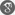##### Cite as:
Ahmed Azzi, Zoubir Hanifi, Mohammed Bekkar, Surfaces as Graphs of Finite Type in H2 × R, Int. J. Anal. Appl., 18 (5) (2020), 838-848.

#### Abstract

In this paper, we prove that ∆X = 2H where ∆ is the Laplacian operator, r = (x, y, z) the position vector field and H is the mean curvature vector field of a surface S in H2 × R and we study surfaces as graphs in H2 × R which has finite type immersion.

#### References

1. M. Bekkar, B. Senoussi, Translation surfaces in the 3-dimensional space satisfying ∆III ri = µiri, J. Geom. 103 (2012), 367-374.2. M. Bekkar, H, Zoubir, Surfaces of revolution in the 3-dimensional Lorentz Minkowski space satisfying ∆j ri = µiri, Int. J. Contemp. Math. Sci. 3 (2008), 1173-1185.3. B-Y. Chen, Total mean curvature and submanifolds of finite type, (2nd edition), World Scientific Publisher, Singapore, 1984.4. F. Dillen, L. Verstraelen, G. Zafindratafa, A generalization of the translation surfaces of Scherk. Differential Geometry in Honor of Radu Rosca: Meeting on Pure and Applied Differential Geometry, Leuven, Belgium, 1989, KU Leuven, Departement Wiskunde (1991), pp. 107–109.5. D. Hoffman, H. Matisse, The computer-aided discovery of new embedded minimal surfaces, Math. Intell. 9 (1987) 8–21.6. P. Scott, The geometries of 3-manifolds, Bull. London Math. Soc. 15 (1983), 401–487.7. B. Senoussi, M. Bekkar, Translation surfaces of finite type in H3 and Sol3, Anal. Univ. Orad. Fasc. Math. Tom, XXVI (1) (2019), 17-29.8. D.W. Yoon, On Translation surfaces with zero Gaussian curvature in H2 ×R, Int. J. Pure Appl. Math. 99 (3) 2015, 289-297.9. D.W. Yoon, Coordinate finite type invariante surfaces in Sol spaces, Bull. Iran. Math. Soc. 43 (2017), 649-658.